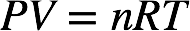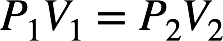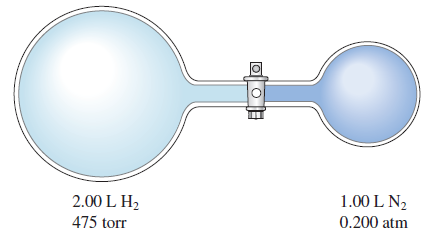# Problem: Consider the flasks in the following diagram. What are the final partial pressures of H 2 and N2 after the stopcock between the two flasks is opened? (Assume the final volume is 3.00 L.) What is the total pressure (in torr)?

🤓 Based on our data, we think this question is relevant for Professor Brunold's class at UW-MADISON.

###### FREE Expert Solution

Recall: The ideal gas law is as follows:We can see that the pressure and volume of a gas are related to the number of moles of gas, the universal gas constant, and the temperature of the gas.

For a given number of moles of gas at a constant temperature, the initial and final pressure and volume of the gas are related by Boyle's law.###### Problem Details

Consider the flasks in the following diagram. What are the final partial pressures of H 2 and N2 after the stopcock between the two flasks is opened? (Assume the final volume is 3.00 L.) What is the total pressure (in torr)?